## 数据说明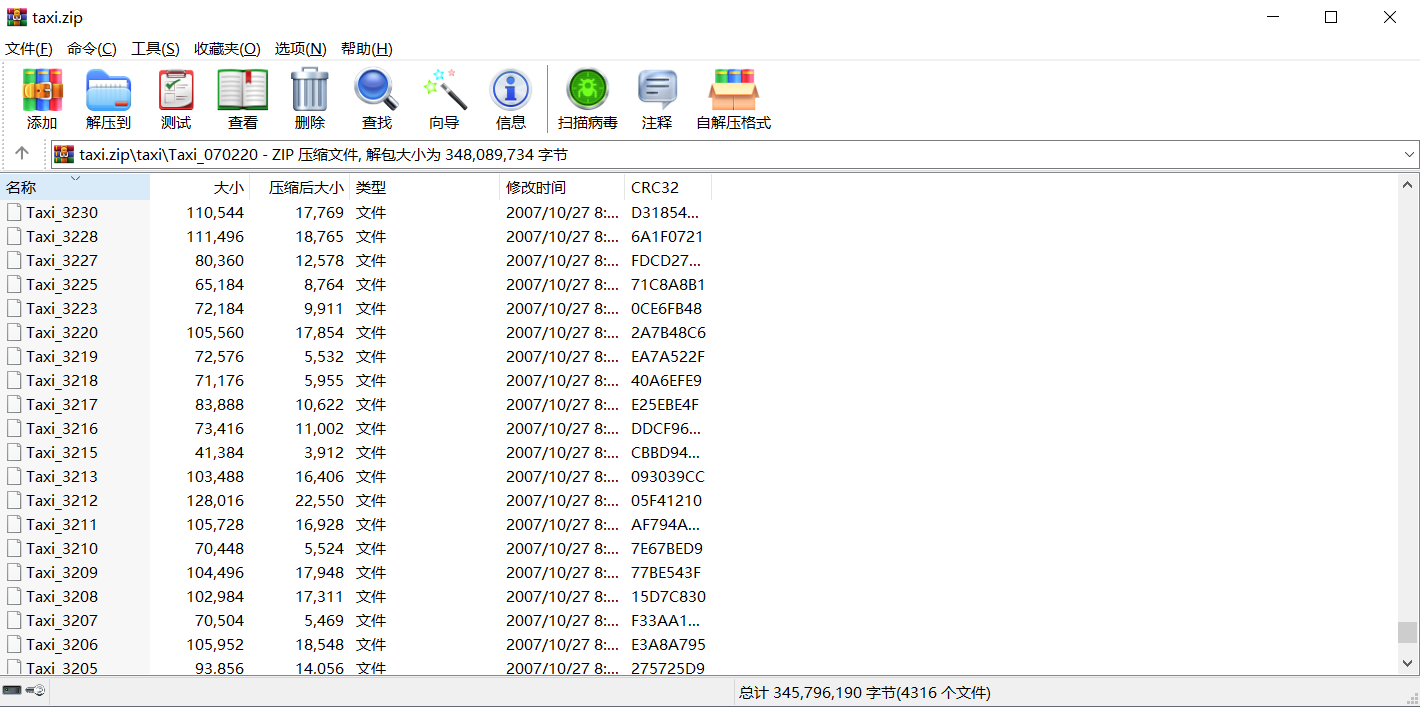## 用xlrd和xlwt读写excel

``````pip install xlwt
``````

``````import os
import xlwt

def eachFile(filepath):
pathDir = os.listdir(filepath)  # 遍历文件夹中的text
return pathDir

fopen = open(name, 'r')
cou = 0
for lines in fopen.readlines():  # 按行读取text中的内容
cou = cou + 1
lines = lines.replace("\n", "").split(",")#分割一下
lines_l = list(lines)#每一行都是一个list了
# print(lines_l)
fopen.close()
return cou

filePath = "G:\\桌面\\Taxi"#装txt的文件夹的路径
pathDir = eachFile(filePath)

ch = []
le = []
le.append(0)
for allDir in pathDir:
# child = os.path.join('%s%s' % (filepath, allDir))
child = "G:\\桌面\\Taxi" + '\\' + allDir
ch.append(child)

#用le记录一个txt有多少行，方便下一个文件读取的时候不会在写入时覆盖
for i in range(1, len(le)):
le[i] = le[i] + le[i - 1]
# print(le)

file = xlwt.Workbook(encoding='utf-8')
# 新建sheet

for i in range(len(ch)):
k = le[i] + 1
print(k)
with open(ch[i]) as f1:
print(ch[i])
lines = lines.replace("\n", "").split(",")
lines_l = list(lines))#每一行都是一个list
i = 0
for line in lines_l:
sheet.write(k, i, line)#一个一个写
i = i + 1
k = k + 1
f1.close()

file.save("G:\\桌面\\taxi.xls")#生成文件的路径，一定要记得save
``````

## 使用openpyxl库读写excel

xlrd和xlwt处理的是xls文件，单个sheet最大行数是65535。我想处理更多数据，网上查到了使用openpyxl函数，最大行数达到1048576。 -----然而我天真的想用这个处理六百万的数据集，跑了几个小时后，文件是生成了，打不开！！如果数据量超过65535就会遇到：ValueError: row index was 65536, not allowed by .xls format最终还是只能存65535行，打开会显示下面的错误。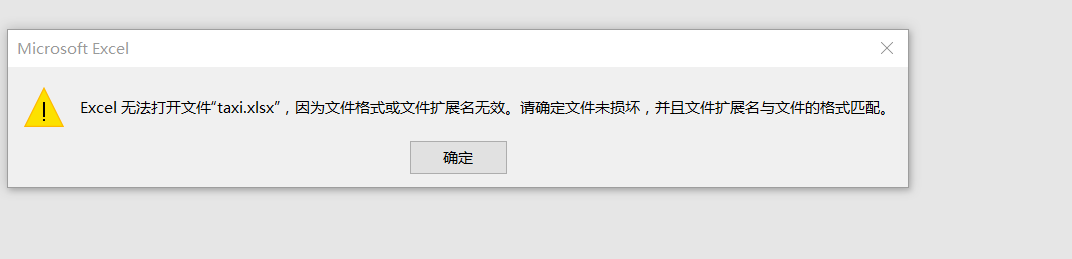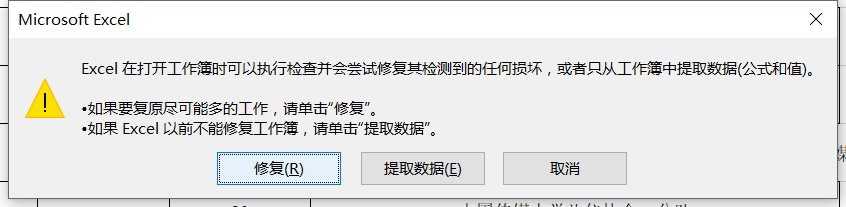``````pip install openpyxl
``````
``````import os
import openpyxl as xl

def eachFile(filepath):
pathDir =os.listdir(filepath)        #遍历文件夹中的text
return pathDir

fopen=open(name, 'r')
cou=0
cou=cou+1
lines = lines.replace("\n", "").split(",")
lines_l = list(lines)
#print(lines_l)
fopen.close()
return cou

filePath = "G:\\桌面\\t_2"
pathDir = eachFile(filePath)

ch = []
for allDir in pathDir:
# child = os.path.join('%s%s' % (filepath, allDir))
child = "G:\\桌面\\t_2" + '\\' + allDir
ch.append(child)

wb = xl.Workbook()
ws = wb.active
ws.title = "sheet"

for i in range(len(ch)):
with open(ch[i]) as f1:
print(ch[i])
lines = lines.replace("\n", "").split(",")
lines_l = list(lines)
ws.append(lines_l)#直接写一行了，比上一个方法简单

f1.close()

wb.save("G:\\桌面\\t_2.xlsx")#这个xlsx跟xls都是excel文件，这个比较新，适用与2013以后版本
``````

## 最简单的批量合并txt（适用海量数据）

### win10批量合并办法：

1、想要进行批量合并首先要将合并的txt文件移动到同一文件夹下，文件名要有顺序，我们善用批量改名功能。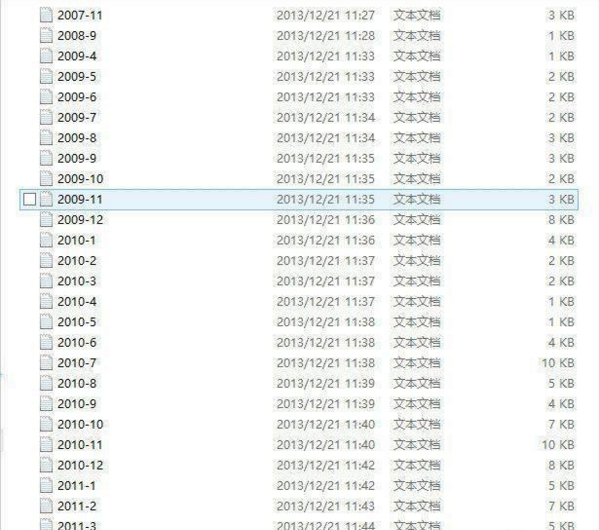2、进入桌面，按下“win + R”快捷键点击运行窗口，写入“cmd”并打开确定点击命令提示符。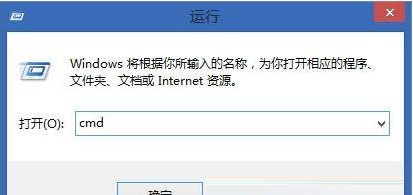3、进入命令提示符窗口后，使用“cd 文件路径”命令进入需要合并的txt文件的目录，小编这里合并的文件是在“F:\stock”目录下。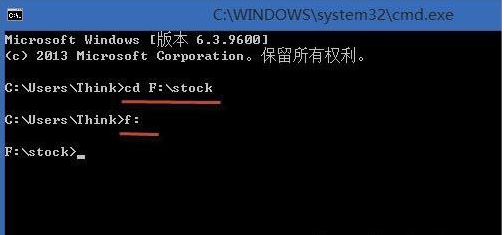4、进入目录后，写入“type *.txt >>f:\111.txt”，按下回车，以后会将当前目录下的所有txt文件的内容按照序号顺序合并到到“f:\111.txt”中。